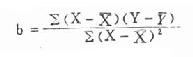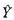二、直线回归方程的计算法 《医学统计学》

# 二、直线回归方程的计算法

1．列回归计算表（见表9.1），计算∑X、∑Y、∑X2、∑Y2、∑XY。

2．计算X、Y、∑(X-X)2、∑(X－X)(Y-Y)

X=∑X/n=15.1/15=1.01

Y=∑Y/n=222/15=14.80

∑(X-X)2=∑X2-(∑X)2/n=0.2093

∑(X-X)(Y-Y)=∑XY-∑X・∑Y/n=-1.7800

3．计算回归系数b和截距a。b和a两值计算公式均是根据最小二乘法的原理推算出来的，其公式如下：（9.5）

a=Y-bX　　　　　　　　（9.6）

a=14.80-(-8.5045)(1.01)=23.3895

4．列出回归方程，绘制回归直线，将求得的b和a的值代入到式（9.4），即得所求的回归方程：=23.3895-8.504X

1=23.3895-8.5045×0.8=16.59,

X2=1.2　则2=23.3895-8.5045×1.2=13.18。

 上一页：一、直线回归方程的意义 当前页：二、直线回归方程的计算法 下一页：三、直线回归方程的假设检验